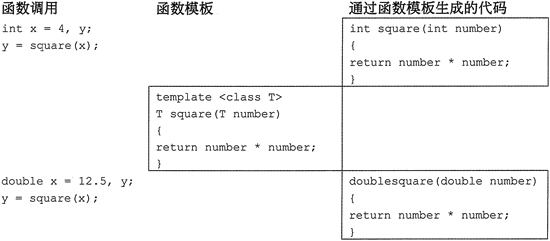## C++函数模板声明和实现

• 内容
• 评论
• 相关

```int square(int number)
{
return number * number;
}
double square(double number)
{
return number * number;
}```

```template<class T>
T square(T number)
{
return number * number;
}```

T square(T number)

int y, x = 4;
y = square(x);

```int square(int number)
{
return number * number;
}```

double y, d = 6.2;
y = square(d);

```double square(double number)
{
return number * number;
}```

```// This program uses a function template.
#include <iostream>
#include <iomanip>
using namespace std;

// Template definition for square function
template <class T>
T square(T number)
{
return number * number;
}

int main()
{
cout << setprecision(5);
//Get an integer and compute its square
cout << "Enter an integer: ";
int iValue;
cin >> iValue;
// The compiler creates int square(int) at the first occurrence of a call to square with an int argument
cout << "The square is " << square(iValue);
// Get a double and compute its square
cout << "\nEnter a double: ";
double dValue;
cin >> dValue;

// The compiler creates double square(double)at the first
// occurrence of a call to square with a double argument
cout << "The square is " << square (dValue) << endl;
return 0;
}```

Enter an integer: 3
The square is 9
Enter a double: 8.3
The square is 68.890条评论